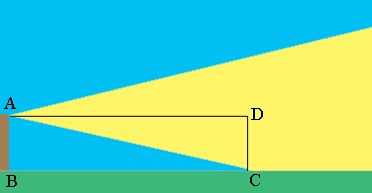SEARCH HOMEMath Central Quandaries & QueriesQuestion from Andrew: If an antenna is atop a building 70 feet tall and the antenna has a beam width of 30 degrees how far from the building does the beam first touch the ground?Hi Andrew,

I drew a diagram of want you described.$AD$ is parallel to the ground and $CD$ is perpendicular to the ground so that triangle $CDA$ is a right triangle. $|AB| = |DC| = 70$ feet and the measure of the angle $DAC$ is $\large \frac{30}{2} \normalsize = 15$ degrees. $|AD| = |BC|$ is the length required.

What trig function relates the measure of the angle $DAC$ to $|DA|$ and $|CD|?$

PennyMath Central is supported by the University of Regina and the Imperial Oil Foundation.